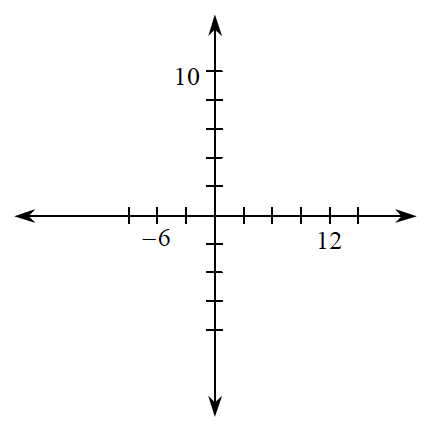### Home > CCAA > Chapter 2 Unit 2 > Lesson CC2: 2.1.2 > Problem2-29

2-29.

Copy the graphs below onto your paper. Then complete the scale by labeling the remaining tick marks.

Scaling Axes

The numbers on each axis of a graph or a number line show the scaling of the axes. The difference between consecutive markings tells the size of the interval. When you scale each axis, you must use equal intervals to represent the data accurately. For example, an interval of $5$ creates a scale numbered $−15$, $−10$, $−5$, $0$, $5$, $10$, $15$, etc. Unequal intervals distort the relationship in the data.

Notice on the graph at right that $80$ marks the end of the fourth interval from zero. If you divide $80$ years by $4$ you can see the length of an interval on this graph is $20$.
$80\div4=20$
The second graph at right has each interval labeled. Labeling the graph this way is called “scaling the axis.”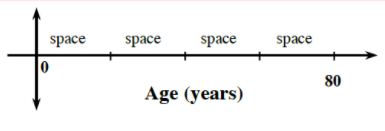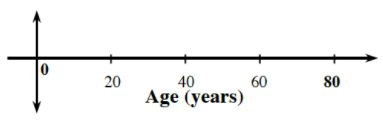1.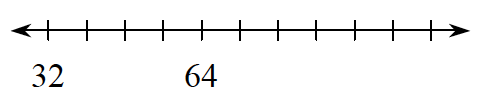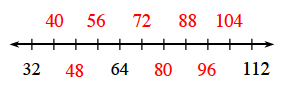1.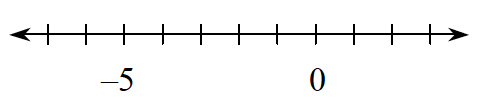1.